# Zener Diodes

## Discrete Semiconductor Devices and Circuits

• #### Question 1

 Don’t just sit there! Build something!!

Learning to mathematically analyze circuits requires much study and practice. Typically, students practice by working through lots of sample problems and checking their answers against those provided by the textbook or the instructor. While this is good, there is a much better way.

You will learn much more by actually building and analyzing real circuits, letting your test equipment provide the “answers” instead of a book or another person. For successful circuit-building exercises, follow these steps:

1. Carefully measure and record all component values prior to circuit construction, choosing resistor values high enough to make damage to any active components unlikely.
2. Draw the schematic diagram for the circuit to be analyzed.
3. Carefully build this circuit on a breadboard or other convenient medium.
4. Check the accuracy of the circuit’s construction, following each wire to each connection point, and verifying these elements one-by-one on the diagram.
5. Mathematically analyze the circuit, solving for all voltage and current values.
6. Carefully measure all voltages and currents, to verify the accuracy of your analysis.
7. If there are any substantial errors (greater than a few percent), carefully check your circuit’s construction against the diagram, then carefully re-calculate the values and re-measure.

When students are first learning about semiconductor devices, and are most likely to damage them by making improper connections in their circuits, I recommend they experiment with large, high-wattage components (1N4001 rectifying diodes, TO-220 or TO-3 case power transistors, etc.), and using dry-cell battery power sources rather than a benchtop power supply. This decreases the likelihood of component damage.

As usual, avoid very high and very low resistor values, to avoid measurement errors caused by meter “loading” (on the high end) and to avoid transistor burnout (on the low end). I recommend resistors between 1 kΩ and 100 kΩ.

One way you can save time and reduce the possibility of error is to begin with a very simple circuit and incrementally add components to increase its complexity after each analysis, rather than building a whole new circuit for each practice problem. Another time-saving technique is to re-use the same components in a variety of different circuit configurations. This way, you won’t have to measure any component’s value more than once.

• #### Question 2

Shown here is the characteristic curve of a diode: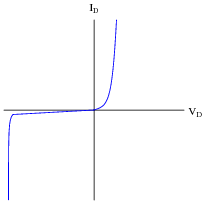Identify which area of this curve represents normal forward-biased operation, and which represents reverse-biased operation. Also, explain the significance of the near-vertical portion of the curve in the lower-left quadrant of the graph.

• #### Question 3

How does the behavior of a zener diode differ substantially from that of a normal (rectifying) diode?

• #### Question 4

Not all “zener” diodes break down in the exact same manner. Some operate on the principle of zener breakdown, while others operate on the principle of avalanche breakdown. How do the temperature coefficients of these two zener diode types compare, and how are you able to discern whether a zener diode uses one principle or the other just from its breakdown voltage rating?

Correspondingly, is there a way we could determine the type of breakdown action from experimental measurements on a zener diode? Explain how such an experiment might be set up.

• #### Question 5

Explain how the characteristic curve of a 24 volt zener diode (as plotted by a curve tracer) differs from that of a normal rectifying diode, shown here: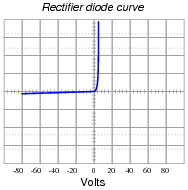• #### Question 6

Suppose you had the boring job of manually maintaining the output voltage of a DC generator constant. Your one and only control over voltage is the setting of a rheostat: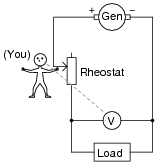What would you have to do to maintain the load voltage constant if the load resistance changed so as to draw more current? Being that your only control over load voltage is the adjustment of a variable resistance in series with the generator, what does this imply about the generator’s output voltage (directly across the generator terminals), compared to the target load voltage?

• #### Question 7

Suppose you had the boring job of manually maintaining the output voltage of a DC generator constant. Your one and only control over voltage is the setting of a rheostat: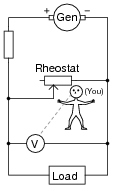What would you have to do to maintain the load voltage constant if the load resistance changed so as to draw more current? Being that your only control over load voltage is the adjustment of a variable resistance in parallel with the load, what does this imply about the generator’s output voltage (directly across the generator terminals), compared to the target load voltage?

• #### Question 8

Describe how a zener diode is able to maintain regulated (nearly constant) voltage across the load, despite changes in load current:• #### Question 9

Calculate the current through the zener diode for the given values of load resistance in this circuit: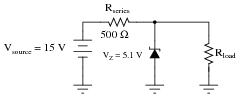Rload = 1 kΩ ; Izener =
Rload = 910 Ω ; Izener =
Rload = 680 Ω ; Izener =
Rload = 470 Ω ; Izener =
Rload = 330 Ω ; Izener =

Do you see any relationship between load current and zener diode current? If so, explain what that relationship is.

• #### Question 10

Calculate the current through the zener diode for the given values of load resistance in this circuit: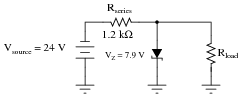Rload = 1.5 kΩ ; Izener =
Rload = 1 kΩ ; Izener =
Rload = 910 Ω ; Izener =
Rload = 780 Ω ; Izener =
• #### Question 11

Calculate the current through the zener diode for the given values of load resistance in this circuit: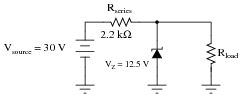Rload = 2 kΩ ; Izener =
Rload = 3 kΩ ; Izener =
Rload = 4 kΩ ; Izener =
Rload = 5 kΩ ; Izener =
• #### Question 12

Calculate the power dissipated by the 5-volt zener diode for the following values of motor current (assume the battery voltage remains constant at 12 volts):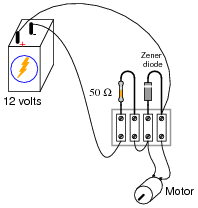Imotor = 20 mA ; Pzener =
Imotor = 50 mA ; Pzener =
Imotor = 90 mA ; Pzener =
Imotor = 120 mA ; Pzener =
Imotor = 150 mA ; Pzener =
• #### Question 13

Calculate the current through the zener diode for the given values of input (source) voltage in this circuit: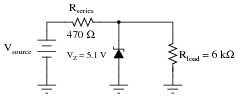Vsource = 25 V ; Izener =
Vsource = 20 V ; Izener =
Vsource = 15 V ; Izener =
Vsource = 10 V ; Izener =
Vsource = 5 V ; Izener =

Do you see any relationship between source voltage and zener diode current? If so, explain what that relationship is.

• #### Question 14

Qualitatively determine what will happen to the series resistor current and the zener diode current in this voltage regulator circuit if the load current suddenly decreases. Assume that the zener diode’s behavior is ideal; i.e. its voltage drop holds absolutely constant throughout its operating range.IRseries = (increase, decrease, or unchanged?)

Izener = (increase, decrease, or unchanged?)

• #### Question 15

Qualitatively determine what will happen to the load current and the zener diode current in this voltage regulator circuit if the source voltage suddenly increases. Assume that the zener diode’s behavior is ideal; i.e. its voltage drop holds absolutely constant throughout its operating range.Iload = (increase, decrease, or unchanged?)

Izener = (increase, decrease, or unchanged?)

• #### Question 16

Zener diodes are simple and useful devices for building voltage regulator circuits, but are times when you may have to improvise in the absence of the proper zener diode. Explain how normal diodes might be used as crude substitutes for a zener diode in the following circuit: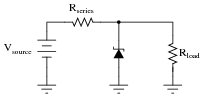• #### Question 17

Suppose you needed to build a simple voltage regulator circuit with a regulation point of 4.5 volts, but had no zener diodes to work with. Can you think of a way normal diodes could be used for the purpose instead?

• #### Question 18

At what load resistance value will this voltage regulator circuit begin to lose its ability to regulate voltage? Also, determine whether the voltage regulation is lost for load resistance values greater than this threshold value, or less than this threshold value.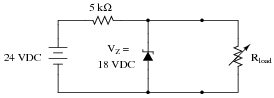• #### Question 19

Prior to the advent of zener diodes, gas-discharge tubes and bulbs were commonly used as voltage regulating devices.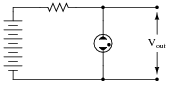Explain how such a device regulates voltage, and comment on whether or not this type of device is still practical in modern circuit design.

• #### Question 20

Precision voltage reference regulators are often constructed of two zener diodes connected in series like this: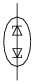Explain why two zener diodes provide greater stability than a single zener diode would, and also draw a circuit showing a voltage source, so this component functions as a complete voltage reference.

• #### Question 21

It is possible to purchase oven-stabilized zener ICs (integrated circuits). Explain what these are, and what they are useful for.

• #### Question 22

How is the parameter of zener impedance defined for a zener diode? Should an ideal zener diode have a zener impedance figure equal to zero, or infinite? Why?

• #### Question 23

Find one or two real zener diodes and bring them with you to class for discussion. Identify as much information as you can about your diodes prior to discussion:

Polarity (which terminal is cathode and which is anode)
Forward voltage drop
Continuous current rating
Surge current rating
Continuous power rating
Zener voltage rating